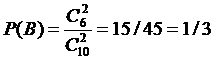Applications
 Home | Definitions | Properties | Interpretations | Gambling | Software | Books

Below are some solved simple exercises and applications:

1)  Find the probability of getting a multiple of 2 at a die roll.
Solution: The number of outcomes that are favorable to respective event is three (these are: {2}, {4}, {6}).  The number of equally possible outcomes is six, so the probability is 3/6 = 1/2 = 50%.

2)  There are three pairs of socks of different colors in a basket. Two socks are randomly extracted from the basket. What is the probability of getting two socks of same color?
Solution:  The number of equally possible cases is the number of all 2-size combinations of socks, namely, C(6, 2) = 15. The number of favorable cases is three, because we have three pairs of socks having the same color. Thus, the probability is  3/15 = 1/5 = 20%.

3)  An urn contains four white balls and six black balls. Two balls are drawn simultaneously. Find the probability of the events: a)  A – drawing two white balls;  b)  B – drawing two black balls;  c)  C – drawing two balls of the same color.
Solution: The number of possible cases is C(10, 2). a)  The number of cases that are favorable to event A is C(4, 2); therefore,. b)  Similarly,. c)  We have. The events A and B are incompatible, so.

4)  Two dice, one red and one blue, are rolled. Consider the events: A – occurrence of a number less than 4 on the red die;  B – occurrence of a number less than 3 on the blue die. Find P(A or B).
Solution:  The cases that are favorable to A are {1}, {2} and {3}; therefore, P(A) = 3/6. The cases that are favorable to B are {1} and {2}; therefore, P(B) = 2/6. The cases that are favorable to A and B correspond to the ordered pairs (1, 1), (1, 2), (2, 1), (2, 2), (3, 1), (3, 2), totalling six, in a probability field where the number of equally possible cases is 6 x 6 = 36. We then have P(A and B) = 6/36. The requested probability is.

5)  At a blackjack game, calculate the probability for a player to get a total of twenty points from the first two cards (provided no other cards are shown), if a 52-card deck is used.
Solution:  The variants totaling twenty points are of the type A + 9 or 10 + 10 (as a value; that is, any 2-size combination of cards from 10, J, Q, K). We have sixteen variants A + 9 (4 aces and 4 nines) and C(16, 2) = 120 variants 10 + 10 (all 2-size combinations of cards from the sixteen cards with a value of 10). The number of all possible distribution variants for two cards is C(52, 2)=1326. The probability is then P = (16 + 120)/1326 = 68/663.

6)  We have two urns, the first containing three white balls and four black balls and the second three white balls and five black balls. A ball is drawn from a randomly chosen urn. Find the probability for the drawn ball to be white.
Solution:  Denote the events: A – the first urn is the chosen one; B – the second urn is the chosen one; C – the drawn ball is white. A and B form a complete system of events and P(A) = P(B) = 1/2. We have  P(CA) = 3/7 and  P(CB) = 3/8.  According to total probability formula, we have: P(C) = P(A)P(CA) + P(B)P(CB) = (1/2) x (3/7) + (1/2) x (3/8) = 45/112 = 0.40178.

7)  Five cards are drawn at once from a 32-card deck, containing cards from 7's up to aces. What is the probability of the five cards containing at least one queen (Q)?
Solution:  Denoting by A the event to be measured the five extracted cards contain at least one Q, we then calculate the probability of the contrary eventthe five extracted cards contain no Q.The equally possible elementary events are the occurrences of 5-size combinations of cards from the 32, a total of C(32, 5). The combinations that are favorable to eventhave the form (xyztv), with x, y, z, t, v taking any card as value, except the four Q-cards. They total C(32 – 4, 5) = C(28, 5). We then have:.

For a broad range of applications in gambling, see the gambling page.

 Sources In the book UNDERSTANDING AND CALCULATING THE ODDS: Probability Theory Basics and Calculus Guide for Beginners, with Applications in Games of Chance and Everyday Life, the teaching material is structured for developing probability calculus skills based on algorithmic procedures. This is the subject of the chapter titled Beginners’ Calculus Guide, in which the reader is taught to apply the properties of probability and to perform calculations in practical applications. The skills acquired here can be practiced on the more than 200 solved and unsolved problems and exercises in the book, whose difficulty level grows gradually.  You may find it in the Books section with a free sample.Software - Books - Contact us